##Hyperbola

 The Hyperbola can be defined by the property of its points X(x,y): ||XF'|-|XF|| = 2a, a being a constant and {F,F'} being fixed points, called the focal points of the hyperbola. Line FF' is called the major axis. The middle O of segment FF' is the center of the hyperbola. Points {A,A'} in which the hyperbola intersects the major axis are called vertices of the hyperbola. The computations below assume that the major axis coincides with the x-axis of the coordinate system and O coincides with the origin of coordinates.
 a = |AA'|/2 is called also the major axis of the hyperbola.
 O is the middle of AA', the circle c centered at O, with radius a is called the auxiliary circle of the ellipse.
 Defining the focal distance, |FF'| = 2c we see easily that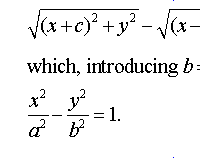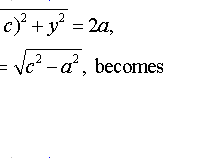b is called the minor axis of the hyperbola. The quantity e = c/a is called the eccentricity of the hyperbola.
 Equation y2/b2 - x2/a2 = 1 defines also a hyperbola called the conjugate hyperbola of the first one.
 The two lines defined by the equation x2/a2 - y2/b2 = 0 are called the assymptotes of the hyperbola.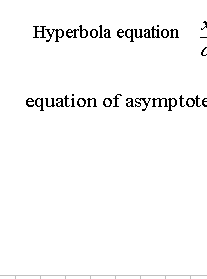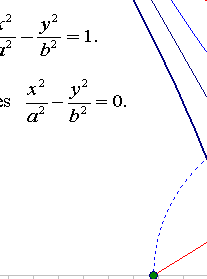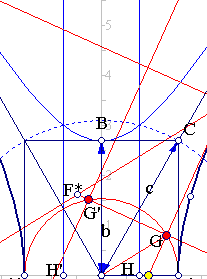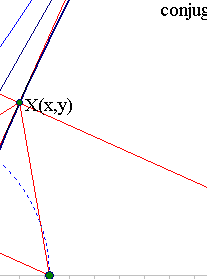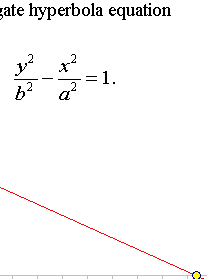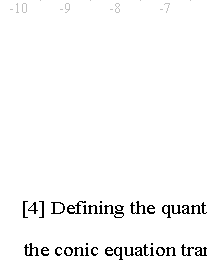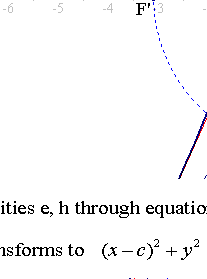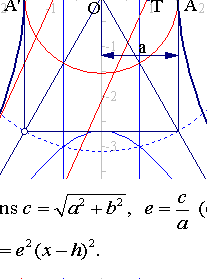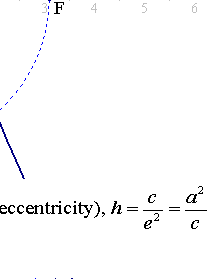The line orthogonal to x-axis at H(h,0) is called a directrix of the hyperbola. Last equation shows that the hyperbola is the locus of points X such that the ratio of distances from focus F and the directrix is equal to e = c/a > 1. The same result holds for the other focus F' and the corresponding directrix, which is the symmetric line to the previous with respect to O. The two directrices are the polars of the foci with respect to the conic.
 The direction of the normal at X(x,y) is (x/a2, -y/b2), hence the equation of the tangent is xu/a2 - yv/b2 = 1 (variable (u,v)). The points {N,T} where the normal/tangent intersect the x-axis are easily calculated to be at {x(a2+b2)/a2, a2/x}, their product being c2=a2+b2. This implies that {T,N} are harmonic conjugate with respect to {F,F'} and since the angle at X is a right one lines XT, XN coincide with the bisectors of the angle FXF'.
 This implies that the symmetric F* of F with respect to the tangent XT is on XF' and the middle G of FF* is on the auxiliary circle with diameter AA'.
 By the symmetry of the hyperbola about its center follows that the other point G' of intersection of line FG with the auxiliary circle has also the property that the normal to FG at G' is a tangent to the hyperbola. This tangent coincides with the tangent at the symmetric point of X i.e. the point with coordinates (-x,-y).
 All properties of hyperbolas, discussed so far, have their twins for ellipses (see Ellipse.html ). Ellipses and hyperbolas are the two kinds of conics called collectively central conics, because they have a center of symmetry.
Look at HyperbolaAsymptotics.html for the equation of the hyperbola with respect to its two (non-orthogonal in general) asymptotic lines.

AntiparallelHyperbola.html
AsymptoticTriangle.html
AsymptoticTriangleInv.html
ConicsMaclaurin.html
Ellipse.html
HyperbolaFromRectangular.html
HyperbolaAsymptotics.html
HyperbolaProperty.html
HyperbolaRectangular.html
HyperbolaWRAsymptotics.html
RectHyperbola.html
RectHypeRelation.html
RectHyperSimpleGeneration.html